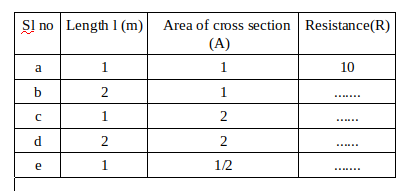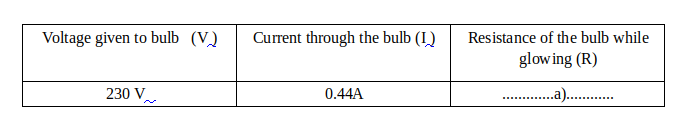Question Pool Current Electricity

# Current Electricity - SAMAGRA Question Pool & Answers | Class 9 English Medium

Kerala Syllabus SAMAGRA SCERT SAMAGRA Question Pool for Class 9 English Medium Physics Current ElectricityQn 1.

Q is the midpoint of the resistor AB. If 4A current flowed through the resistor when the switch was turned on as shown in the figure, then

a)If the point S touches the midpoint Q of AB, what will be the current in the circuit?

b)In the same circuit, if the point A is connected to the point B and S touches the midpoint Q of AB, then what will change the current?

c)If the conductor AB is stretched and doubled in length, will the current in the circuit be changed?

a) When V / I = R; V / R = I

when S touches the midpoint Q, R is reduced by half, V / R / 2 = I; V / R = 2I The electric current doubles I = 8A

b)

Get Free Study Materials + 1 Week Free Trial of BrainsPrep Class 9 English Medium Tuition

Qn 2.

Identify the relation in the first pair and complete the second pair

Current is formed in conductors ; By the flow of free electrons

Current is formed in electrolytes and gases; ...................................

By the flow of

Get Free Study Materials + 1 Week Free Trial of BrainsPrep Class 9 English Medium Tuition

Qn 3.

Which among the following doesn’t influence the resistance of a conductors

(length, Area of cross section , density, resistivity)

Get Free Study Materials + 1 Week Free Trial of BrainsPrep Class 9 English Medium Tuition

Qn 4.

.A table related to the resistance of a conductor is given. Complete the tablea- 20ohm

b-5 ohm

c-10

Get Free Study Materials + 1 Week Free Trial of BrainsPrep Class 9 English Medium Tuition

Qn 5.

.Instrument used to regulate the current in a circuit by gradually changing the resistance is ------

Get Free Study Materials + 1 Week Free Trial of BrainsPrep Class 9 English Medium Tuition

Qn 6.

Some symbols are given in the column A. Find out the suitable names from column B for each one and write against it.

IMAGE

a. cell b- Resistance, c-Bulb, d- Switch , e-

Get Free Study Materials + 1 Week Free Trial of BrainsPrep Class 9 English Medium Tuition

Qn 7.

The resistance of a bulb is 42Ω. The current  passed through the conductor when 230 V supplied is given in the table.

Complete the tableb) Will the resistance of the filament be same when the bulb is on and off? What could be the reason?

a) R=522.7Ω

b) No. When temperature increases the resistance also

Get Free Study Materials + 1 Week Free Trial of BrainsPrep Class 9 English Medium Tuition

Qn 8.

Find out the relation from the first pair and complete the second pair

kgm/s2 : N

Volt/ Ampere : .............

Ohm

Get Free Study Materials + 1 Week Free Trial of BrainsPrep Class 9 English Medium Tuition

Qn 9.

0.2 A current is flowing through a soldering iron having resistance 500Ω .Find out the voltage between the ends of the soldering iron.

According to Ohms law,V = IxR

= 500x0.2=

Get Free Study Materials + 1 Week Free Trial of BrainsPrep Class 9 English Medium Tuition

Qn 10.

10A current is flowing through a heater working in 230V. Calculate the resistance of the heating coil.

According to Ohms Law V/I=R

Get Free Study Materials + 1 Week Free Trial of BrainsPrep Class 9 English Medium Tuition

Qn 11.

20 coulomb charge passed in 5 seconds through a circuit when connected to a 12 volt battery.

a) What is the intensity of current through the circuit?

b) Which instrument is used to measure the Intensity of current?

c)Draw a circuit by including thid device.

Get Free Study Materials + 1 Week Free Trial of BrainsPrep Class 9 English Medium Tuition

Qn 12.

Find the relation from the first pair and complete the second pair

Potential difference : Voltmeter

Intensity of current : ...............................

Get Free Study Materials + 1 Week Free Trial of BrainsPrep Class 9 English Medium Tuition

Qn 13.

Find the relation from the first pair and complete the second pair

Potential difference : Volt (V)

Intensity of current : ...................

Get Free Study Materials + 1 Week Free Trial of BrainsPrep Class 9 English Medium Tuition

Qn 14.

Calculate the intensity of current if a charge of 40 coulomb passes through a circuit in 8 seconds.

I  =40/8=

Get Free Study Materials + 1 Week Free Trial of BrainsPrep Class 9 English Medium Tuition

Qn 15.

Draw a circuit to get an effective voltage of 3V using 4 cells of each 1.5 volt.

Get Free Study Materials + 1 Week Free Trial of BrainsPrep Class 9 English Medium Tuition

Qn 16.

Observe the circuit diagram given below and answer the questions.

IMAGE

a) How are the voltmeter and bulb connected in the circuit?

(series/parallel)

b) How will you connect an ammeter in the circuit to measure the current?

a) parallel

b) series

Get Free Study Materials + 1 Week Free Trial of BrainsPrep Class 9 English Medium Tuition

Qn 17.

Cells connected in two different ways are shown below.

IMAGE

a) Find out and write how cells are connected in each circuit.

b) what change will happen to the effective voltage in the two arrangements?

a) series ,

b) parallel

b)

Get Free Study Materials + 1 Week Free Trial of BrainsPrep Class 9 English Medium Tuition

Qn 18.

identify the relation from the first pair and complete the second pair

Generator ; Mechanical energy---- Electrical energy

Cell : ............................ ..........................

Chemical energy .... electrical

Get Free Study Materials + 1 Week Free Trial of BrainsPrep Class 9 English Medium Tuition

Qn 19.

Which among the following are not a source of emf.

( Generator, Electric heater , solar cell, electro chemical cell, Electric motor)

Electric heater, Electric

Get Free Study Materials + 1 Week Free Trial of BrainsPrep Class 9 English Medium Tuition

Qn 20.

if 10J work is done while moving 1 coloumb charge from P to Q ,what is the potential between the points

10V